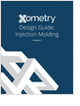MaterialsDesign CenterProcessesUnit ConversionFormulasMathematicsCalculatorsDiscussion ForumTrade PublicationsDirectory Service
Injection Molding Design Guide

Guide for high quality and cost-effective plastic injection molding.

Salary Expectation

8 things to know about the interview question "What's your salary expectation"?

Learn the best principles to negotiate the salary you deserve!

CNC Machining Design Guide

more free publicationsGlossary » Units » Electric Charge Surface Density » Coulomb Per Square CentimeterCoulomb Per Square Centimeter (C/cm2) is a unit in the category of Electric charge surface density. It is also known as coulombs per square centimeter, coulomb/square centimeter, coulomb per square centimetre, coulombs per square centimetre. Coulomb Per Square Centimeter (C/cm2) has a dimension of L-2TI where L is length, T is time, and I is electric current. It can be converted to the corresponding standard SI unit C/m2 by multiplying its value by a factor of 10000.
Note that the seven base dimensions are M (Mass), L (Length), T (Time), Q (Temperature), N (Aamount of Substance), I (Electric Current), and J (Luminous Intensity).

Other units in the category of Electric charge surface density include Abcoulomb Per Square Centimeter (abcoulomb/cm2), Coulomb Per Square Inch (C/in2), and Coulomb Per Square Meter (C/m2).N/ARelated PagesGlossaryInjection Molding Design Guide

Guide for high quality and cost-effective plastic injection molding.Salary Expectation

8 things to know about the interview question "What's your salary expectation"?